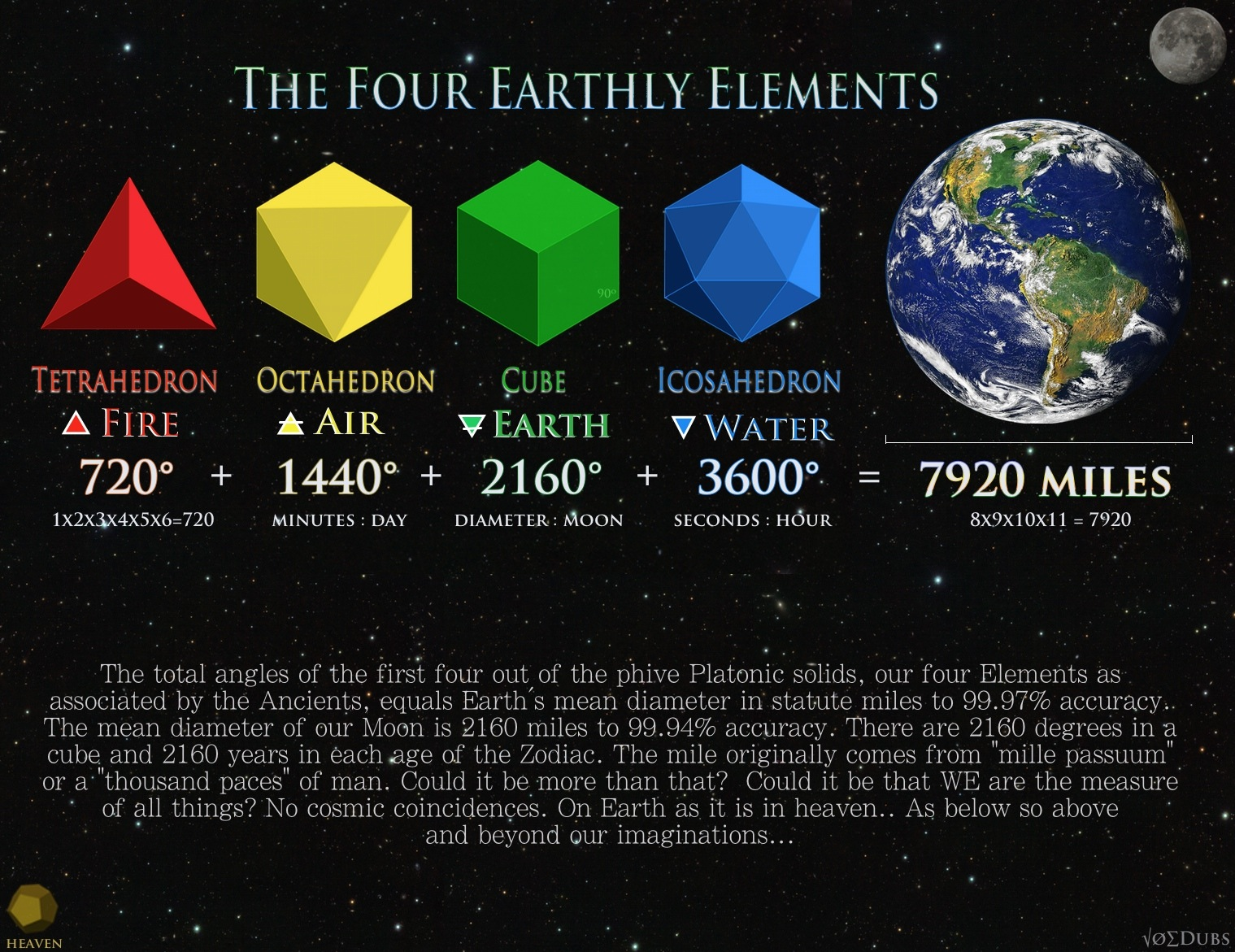## Organizing RealityWhy do we organize things in groups of ten? You could argue that it’s only because we use the decimal system or base-10 mathematics, so it just appears that way.  However there is a lot of evidence that supports the idea that the decimal system is not some made-up anthropocentric contrivance, but a scalable numerical matrix to our reality.  Wikipedia tells us, we use base-10 because it is the most convenient since we have 10 fingers.  But as we’ll explore, there’s much more to it than that. The tetractys is a mystical symbol and was very important to the secret worship of the Pythagoreans.  A triangular figure consisting of ten points arranged in four rows: one, two, three, and four points in each row, which is the geometrical representation of the fourth triangular number.  The Tetragrammaton is the the name of God written in four Hebrew letters usually transliterated YHWH.  Both of…

7

## Sacred Geometry of the MoonThe physical dimensions of Earth and Moon reveal astounding geometries. The sacred geometry of the Moon was understood long ago and the ancients hid the esoteric meaning in the dimensions of their monuments and architecture. The Moon encodes the first two Pythagorean Triples, which are sets of whole numbers that satisfy the equation popularized by Pythagoras that everyone is familiar with. Pythagorean Theorem a^squared + b^squared = c^squared In the book “Taking Measure”, Scott Onstott shows that the point of intersection that defined the amount of full moons in a year (12.369), lies on the perfect fifth (2:3) ratio on the smallest side of the triangle. These numbers also encode esoteric knowledge about the geometric locations of Stonehenge and other sacred sites in England. He continues: “In The Lost Science of Measuring the Earth, John Michell and Robin Heath identified a 5:12:13 Pythagorean triangle…

2

## Mathemagical Synchronicities in our Measure of Space and TimeOur units of measurement of space and time encode a synergistic system, a sacred canon of number beyond that of which we commonly associate today.  Miles, furlongs, minutes, seconds, degrees, these units synchronize with a cosmic order creating a divine system in our holofractographic reality. These are the mathemagical synchronicities in our measure of time and space.  One ft is not one foot because it is the size of a King’s shoe back in the day.  One foot is one ft because it aligns with the cosmos as well of that of man.  As the popular hermetic axiom succinctly phrases it, As above so below.  The ancient philosopher Protagoras put it another way;  “Man is the measure of all things.” “In ancient Greece the advanced students of the philosopher Pythagoras who were engaged in deep studies of natural science and self-understanding were called “mathematekoi”…

4